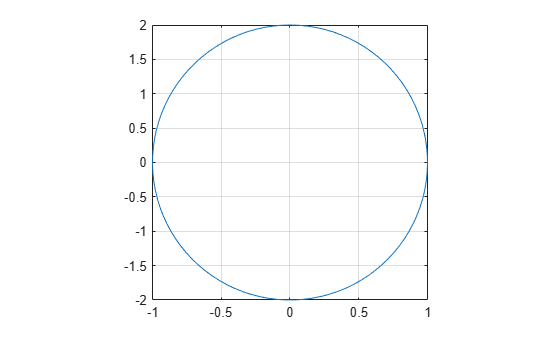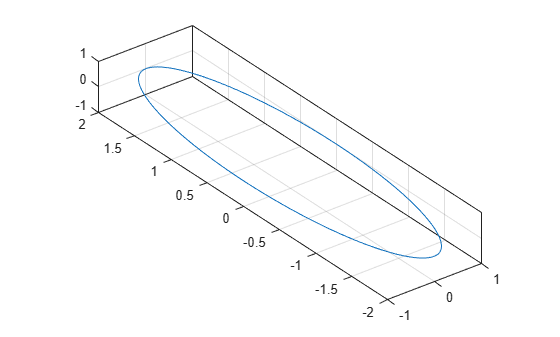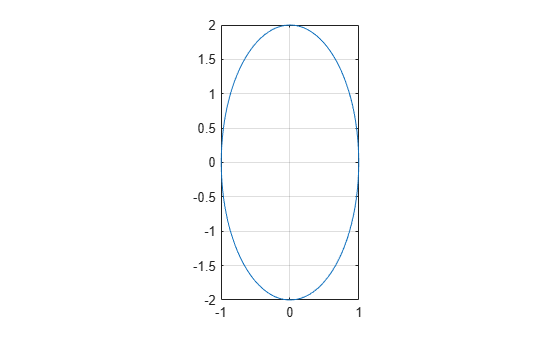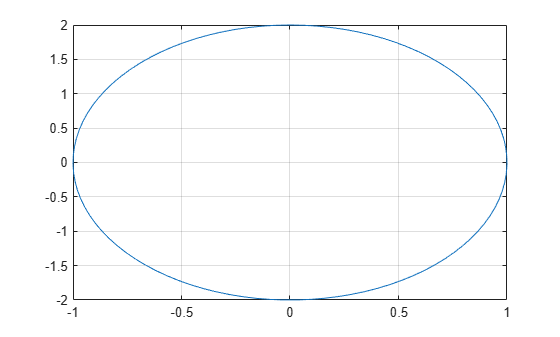Main Content

## Control Ratio of Axis Lengths and Data Unit Lengths

You can control the relative lengths of the x-axis, y-axis, and z-axis (plot box aspect ratio). You also can control the relative lengths of one data unit along each axis (data aspect ratio).

### Plot Box Aspect Ratio

The plot box aspect ratio is the relative lengths of the x-axis, y-axis, and z-axis. By default, the plot box aspect ratio is based on the size of the figure. You can change the aspect ratio using the `pbaspect` function. Set the ratio as a three-element vector of positive values that represent the relative axis lengths.

For example, plot an elongated circle. Then set the plot box aspect ratio so that the x-axis is twice the length of the y-axis and z-axis (not shown).

```t = linspace(0,2*pi); plot(sin(t),2*cos(t)) grid on pbaspect([2 1 1])```Show the axes in a 3-D view to see the z-axis.

`view(3)`For square axes, use `[1 1 1]`. This value is similar to using the `axis square` command.

```t = linspace(0,2*pi); plot(sin(t),2*cos(t)) grid on pbaspect([1 1 1])```### Data Aspect Ratio

The data aspect ratio is the relative length of the data units along the x-axis, y-axis, and z-axis. You can change the aspect ratio using the `daspect` function. Set the ratio as a three-element vector of positive values that represent the relative lengths of data units along each axis.

For example, set the ratio so that the length from 0 to 1 along the x-axis is equal to the length from 0 to 0.5 along the y-axis and 0 to 2 along the z-axis (not shown).

```t = linspace(0,2*pi); plot(sin(t),2*cos(t)) grid on daspect([1 0.5 2])```Show the axes in a 3-D view to see the z-axis.

`view(3)`For equal data units in all directions, use `[1 1 1]`. This value is similar to using the `axis equal` command. One data unit in the x direction is the same length as one data unit in the y and z directions.

```t = linspace(0,2*pi); plot(sin(t),2*cos(t)) grid on daspect([1 1 1])```### Revert Back to Default Ratios

Change the data aspect ratio. Then revert back to the default plot box and data aspect ratios using the `axis normal` command.

```t = linspace(0,2*pi); plot(sin(t),2*cos(t)) grid on daspect([1 1 1]) axis normal```Download ebook